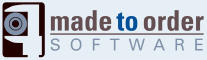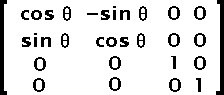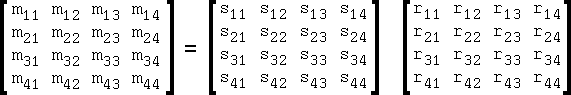# Appendix A — The geometry in SWF — Matrix

The coordinates are often transformed with the use of a matrix. The matrix is similar to a transformation matrix in Postscript. It includes a set of scaling factors, rotation angles and translations.

When only the scaling factors are used (no rotation) then these are ratios as one would expect. If a rotation is also applied, then the scaling ratios will be affected accordingly.

The translations are in TWIPS like any coordinates and also they are applied last (thus, it represents the position at which the shape is drawn on the output screen).

The math formula is as simple as: Q = MP + T. Q is the resulting point, P is the source point, M is the scaling and rotation factors and T is the final translation.

With the use of a three dimensional set of matrices, one can compute a single matrix which includes all the transformations.

 T =Fig 1. Matrix The Tx and Ty are set as defined in the SWF file. Tz can be set to zero. S =Fig 2. Matrix The Sx and Sy are set as defined in the SWF file when no rotation are defined. Sz is always set to 1. Rx =Fig 3. Matrix This matrix shows you a rotation over the X axis. This is not necessary for the SWF format. Ry =Fig 4. Matrix This matrix shows you a rotation over the Y axis. This is not necessary for the SWF format. Rz =Fig 5. Matrix This matrix shows you a rotation over the Z axis. This is used by the SWF format. However, it is mixed with the scaling factors. It is rare not to have a scaling factor when a rotation is applied.

Thus, the matrix saved in the SWF file is the product of the matrix in figure 2 and the matrix in figure 5.Fig 6. Matrix

A matrix multiplication is the sum of the products of rows (left matrix) and columns (right matrix).

```	m11 = s11 * r11 + s12 * r21 + s13 * r31 + s14 * r41

m12 = s21 * r11 + s22 * r21 + s23 * r31 + s24 * r41

m13 = s31 * r11 + s32 * r21 + s33 * r31 + s34 * r41

m14 = s41 * r11 + s42 * r21 + s43 * r31 + s44 * r41

m21 = s11 * r12 + s12 * r22 + s13 * r32 + s14 * r42

m22 = s21 * r12 + s22 * r22 + s23 * r32 + s24 * r42

m23 = s31 * r12 + s32 * r22 + s33 * r32 + s34 * r42

m24 = s41 * r12 + s42 * r22 + s43 * r32 + s44 * r42

m31 = s11 * r13 + s12 * r23 + s13 * r33 + s14 * r43

m32 = s21 * r13 + s22 * r23 + s23 * r33 + s24 * r43

m33 = s31 * r13 + s32 * r23 + s33 * r33 + s34 * r43

m34 = s41 * r13 + s42 * r23 + s43 * r33 + s44 * r43

m41 = s11 * r14 + s12 * r24 + s13 * r34 + s14 * r44

m42 = s21 * r14 + s22 * r24 + s23 * r34 + s24 * r44

m43 = s31 * r14 + s32 * r24 + s33 * r34 + s34 * r44

m44 = s41 * r14 + s42 * r24 + s43 * r34 + s44 * r44
```

Though you shouldn't need to find the scaling factors and rotation angle from an SWF matrix, it is possible to find one if you know the other. This is done using a multiplication of either the inverse scaling (use: `1/Sx` and `1/Sy` instead of `Sx` and `Sy` for the scaling matrix) or the inverse rotation (use: `-angle` instead of `angle` in the Z rotation matrix).

For those who still wonder what I'm talking about, there are the four computations you need from a scaling factor and an angle in radiant:

```	SWFmatrix11 =  Sx * cos(angle)
SWFmatrix12 =  Sy * sin(angle)
SWFmatrix21 = -Sx * sin(angle)
SWFmatrix22 =  Sy * cos(angle)
```

`SWFmatrix11` and `SWFmatrix22` are saved in the (x, y) scale respectively and the `SWFmatrix21` and `SWFmatrix12` are the rotation skew0 and skew1 values respectively.